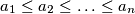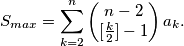### IMO Shortlist 1999 problem A6

Kvaliteta:
Avg: 0,0
Težina:
Avg: 8,0
For$n \geq 3$ and$a_{1} \leq a_{2} \leq \ldots \leq a_{n}$ given real numbers we have the following instructions:

- place out the numbers in some order in a ring;
- delete one of the numbers from the ring;
- if just two numbers are remaining in the ring: let$S$ be the sum of these two numbers. Otherwise, if there are more the two numbers in the ring, replace

Afterwards start again with the step (2). Show that the largest sum$S$ which can result in this way is given by the formulaIzvor: Međunarodna matematička olimpijada, shortlist 1999